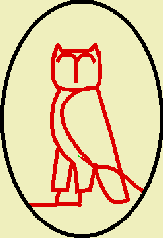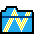# !Pellian

## May 2011!Pellian - Pellian Equation Solver - Version 1.00, 27th May 2011

===============================================

A 'Diophantine' Equation is one to be solved in Whole (non-Fractional) Numbers.

A Special Type of such Equation is the 'Pellian' Equation, which is given by:-

x^2 - D.y^2 = 1, to be solved for some given Whole D, and x and y, Whole.

This problem is related to the 'continued fraction' for the Square Root of D.

A 'continued fraction' is of the form:- a0 + 1/(a1 + 1/(a2 + 1/(a3... etc.

It turns out that the 'continued fraction' for the square root of a (given) Whole Number, D,

starts repeating itself, after a bit. Also, if we terminate the 'continued fraction', when

it starts to repeat, and work back to the resulting fraction - numerator/denominator, THEN

numerator^2 - D.denominator^2 = -1^m, where m is the number of 'terms' in the terminated

fraction. This is called the 'least' solution, call this 'p' and 'q' and p^2-d.q^2 = -1^m.

It also turns out, given p and q, then we can obtain an infinite number of further solutions:-

x = ((p + q.sqt(D))^n + (p - q.sqrt(D))^n)/2.0 and

y = ((p + q.sqt(D))^n - (p - q.sqrt(D))^n)/(2.0 * sqrt(D)), where sqrt(D) is the square root of D.

For n=1, this yields the original p and q for x and y, respectively.

The only difference for m being odd, is that we must make the 'n's even, or else we alternately

get solutions to x^2 - D.y^2 = -1, then +1.

This program finds the 'least' solution, then generates further solutions, until the numbers

get too large to be held in the allocated computer representation. As we vary D, sometimes

there is a relatively 'simple' solution, but sometimes even the 'least' solution, itself, is

too large to be held on the computer. You are warned when this happens. D must not be a square.

Because the small 'least' solutions get rarer, as we increase D, you are limited to D<=300.

### Instructions for Use:-

Unzip the downloaded compressed file, and extract the !Pellian application to a suitable directory (folder) on your hard disc.

Double-clicking on the !Pellian program icon, runs the application and loads its icon onto the icon bar.

After loading the !Pellian application onto the icon bar, left clicking on the bar icon will produce a 'SetUp' panel.

Type the value of the constant, D, in the Pellian Equation into the slot provided.

NB Press the 'Return' (Enter) key after having entered the value to register it.

Clicking 'Calculate', then finds X, and Y values, such that X^2 - D . Y^2 =1, provided D<=300 and not a square.

Menus are available from the bar icon, the 'SetUp' panel, or the 'Help' window. In particular, you may save a Text file with the solutions in it, from the 'SetUp' panel menu.

###Click on Icon to DownLoad Zipped !Pellian Version 1.00 Dated May 27th 2011.

This document was generated on 29th May 2011 for this Acorn RISC OS Software Application written and distributed by Martin Carradus